# w4. 1a) For the following reaction, 0.275 moles of hydrochloric acid are mixed with 0.372 moles of oxygen gas. hydrochlo...

w4.

1a) For the following reaction, 0.275 moles of hydrochloric acid are mixed with 0.372 moles of oxygen gas.

hydrochloric acid (aq) + oxygen (g) --> water (l) + chlorine (g)

What is the formula for the limiting reagent?

What is the maximum amount of water that can be produced? (answer in moles)

1b) An iron nail rusts when exposed to oxygen. For the following reaction, 0.343 moles of iron are mixed with 0.127 moles ofoxygen gas.

iron (s) + oxygen (g) --> iron (III)oxide (s)

What is the formula for the limiting reagent?

What is the maximum amount of iron(III) oxide that can be produced? (answer in moles)

1c) For the following reaction, 0.353 moles of carbon disulfide are mixed with 0.467 moles of chlorine gas.

carbon disulphide (s) + chlorine (g) --> carbon tetrachloride (l) + sulfur dichloride (s)

What is the formula for the limiting reagent?

What is the maximum amount of carbon tetrachloride that can be produced? (answer in moles)

2a) Write a net ionic equation for the reaction that occurs when aqueous solutions of  sodium hydroxide and  hydrobromic acid are combined.

Write whether they are aq, s, l, or g and fill in the spaces below.

__________ + __________ + __________ + __________ --> __________ + __________

2b) Write a net ionic equation for the reaction that occurs when aqueous solutions of hypochlorous acid and sodium hydroxideare combined.

Write whether they are aq, s, l, or g and fill in the spaces below.

__________ + __________ + __________ + __________ --> __________ + __________

2c) Write a net ionic equation for the overall reaction that occurs when aqueous solutions of  carbonic acid and  sodium hydroxide are combined. Assume excess base.

Write whether they are aq, s, l, or g and fill in the spaces below.

__________ + __________ + __________ + __________ --> __________ + __________ + __________ + __________

3a) Write a net ionic equation for the reaction that occurs when aqueous solutions of ammonia and hydrobromic acid are combined.

Write whether they are aq, s, l, or g and fill in the spaces below.

__________ + __________ --> __________ + __________

3b) Write a net ionic equation for the reaction that occurs when aqueous solutions of ammonia and hydrocyanic acid are combined.

Write whether they are aq, s, l, or g and fill in the spaces below.

__________ + __________ --> __________ + __________

3c) Write a net ionic equation for the reaction that occurs when aqueous solutions of hydrochloric acid and ammonia are combined.

Write whether they are aq, s, l, or g and fill in the spaces below.

__________ + __________ --> __________ + __________

4a) A molecule or ion that donates the hydrogen in a hydrogen bond is a hydrogen bond donor

A molecule or ion that binds to this hydrogen is a hydrogen bond acceptor.
Specify the hydrogen bonding behavior of the 4 species below by selecting:
donor for species that act as donors only
acceptor for species that act as acceptors only
both for species that act as both donors and acceptors
neither for species that act neither as donors nor as acceptors.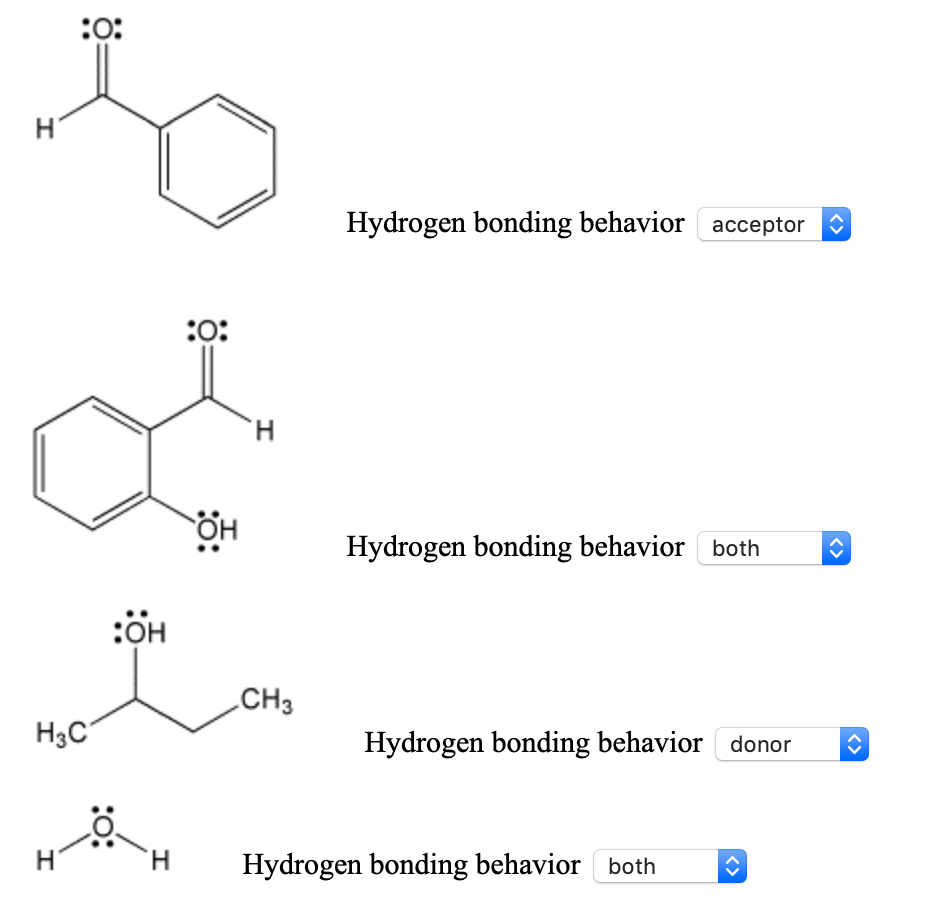4b) A molecule or ion that donates the hydrogen in a hydrogen bond is a hydrogen bond donor
A molecule or ion that binds to this hydrogen is a hydrogen bond acceptor.
Specify the hydrogen bonding behavior of the 4 species below by selecting:
donor for species that act as donors only
acceptor for species that act as acceptors only
both for species that act as both donors and acceptors
neither for species that act neither as donors nor as acceptors.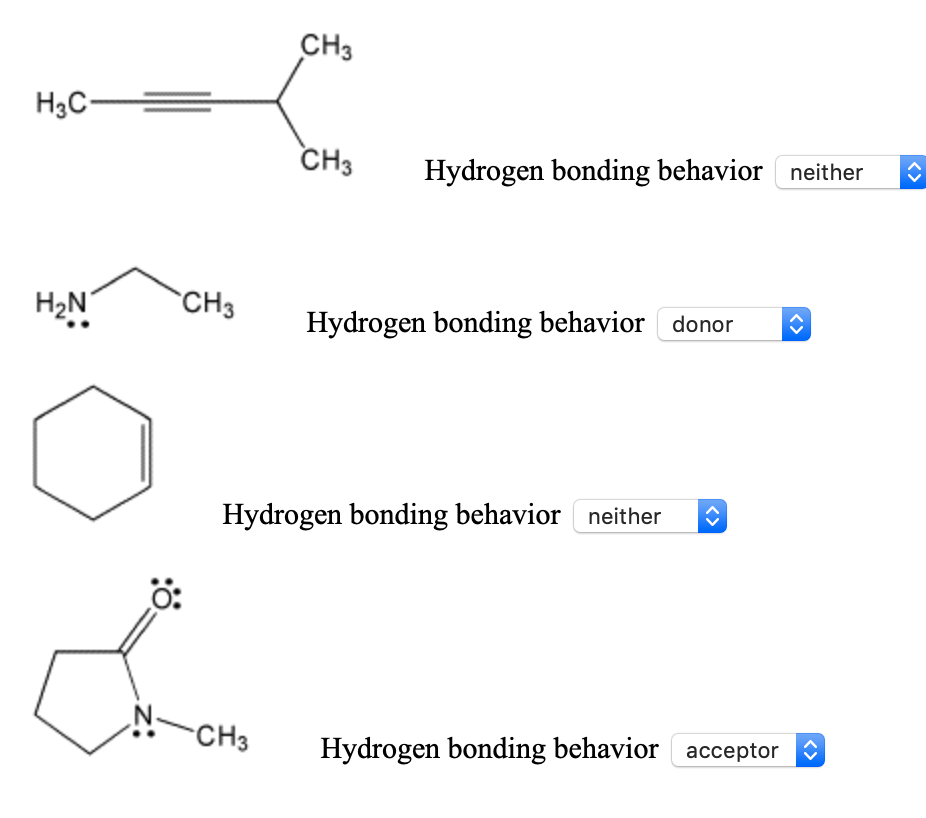w9.

5a) Match the gas sample on the left with a description on the right.
You may use the same answer more than once.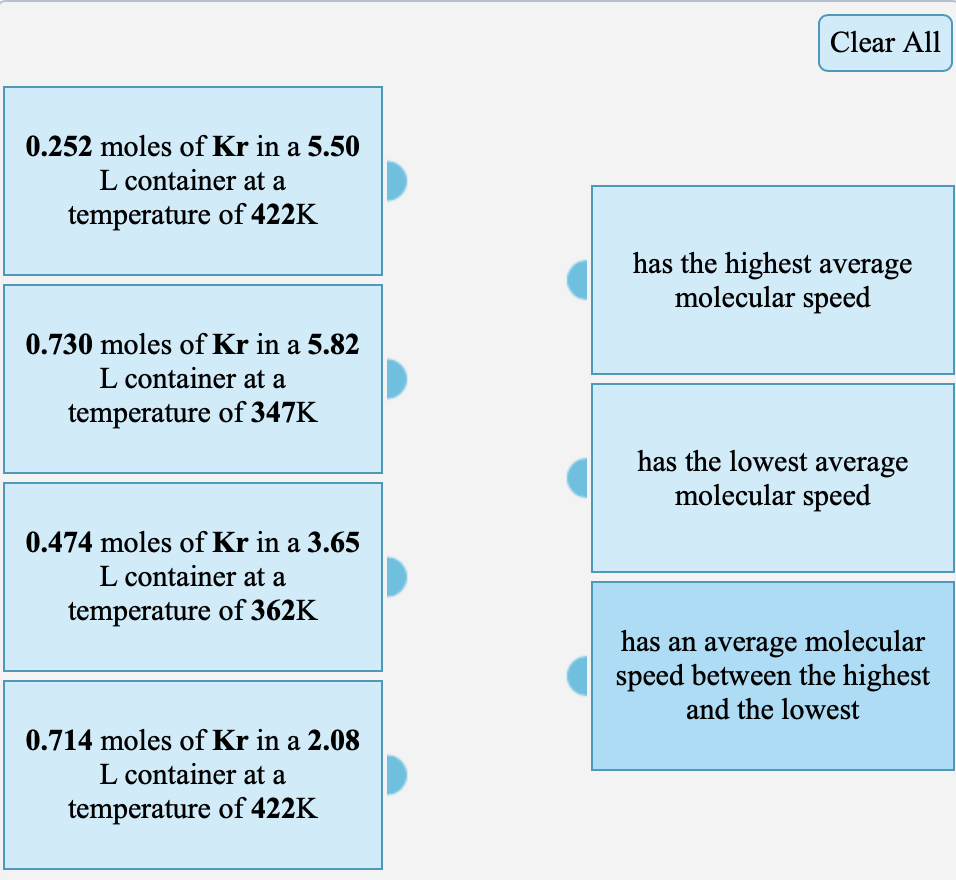5b) Arrange the following molecules in order of increasing average molecular speed. All are at the same temperature.

carbon dioxide, ammonia, krypton, neon

Enter formulas in the boxes below: 1 = slowest, 4 = fastest

5c) Arrange the following molecules in order of increasing average molecular speed.

NO2 at 384K, NH3 at 442K, O2 at 384K, NO2 at 333K

Enter formulas and temperatures in the boxes below:
1 = slowest, 4 = fastest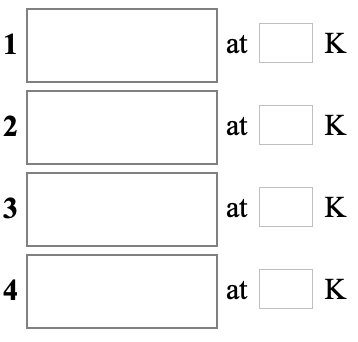6a) A helium-filled weather balloon has a volume of 588 L at 18.9°C and 758 mmHg. It is released and rises to an altitude of8.65 km, where the pressure is 284 mmHg and the temperature is –35.1°C.

The volume of the balloon at this altitude is __________ L.

6b)

A sample of  xenon gas occupies a volume of  6.74 L at  45.0°C and  1.29 atm.

If it is desired to  increase the volume of the gas sample to  9.20 L, while  decreasing its pressure to  0.775 atm, the temperature of the gas sample at the new volume and pressure must be __________°C.

H Hydrogen bonding behavior acceptor н H Hydrogen bonding behavior both :OH CHз Нас Hydrogen bonding behavior donor Hydrogen bonding behaviorboth I
CHз Нас- CHз neither Hydrogen bonding behavior CH3 НaN donor Hydrogen bonding behavior neither Hydrogen bonding behavior -CH3 acceptor Hydrogen bonding behavior
Clear All 0.252 moles of Kr in a 5.50 L container at a temperature of 422K has the highest average molecular speed 0.730 moles of Kr in a 5.82 L container at a temperature of 347K has the lowest average molecular speed 0.474 moles of Kr in a 3.65 L container at a temperature of 362K has an average molecular speed between the highest and the lowest 0.714 moles of Kr in a 2.08 L container at a temperature of 422K
K 1 at K 2 at 3 K at K 4 at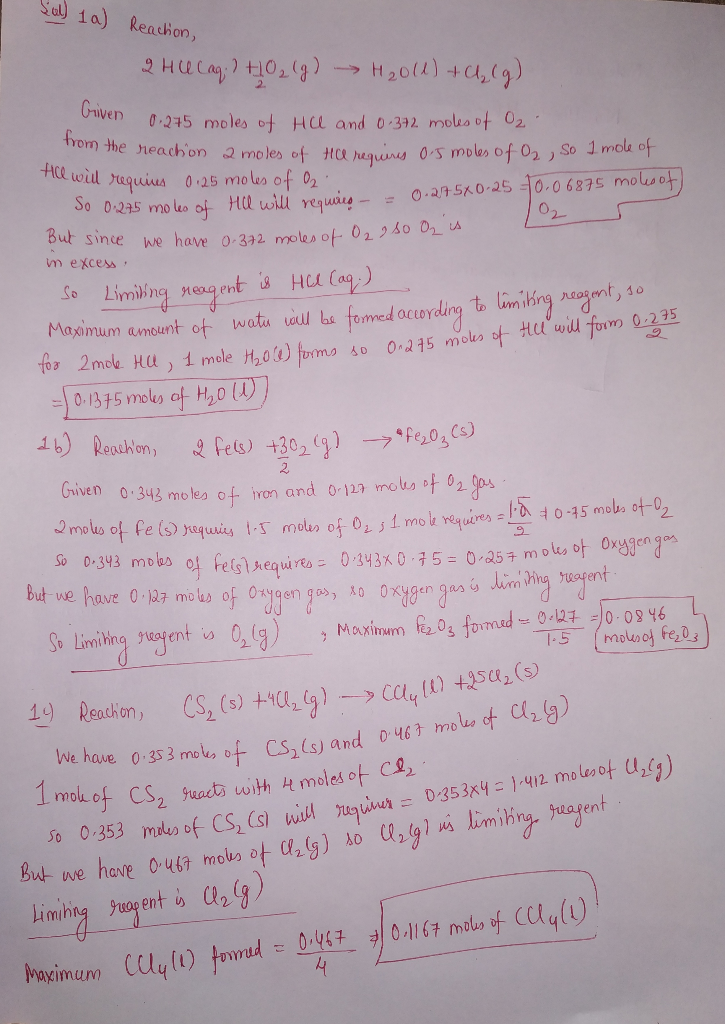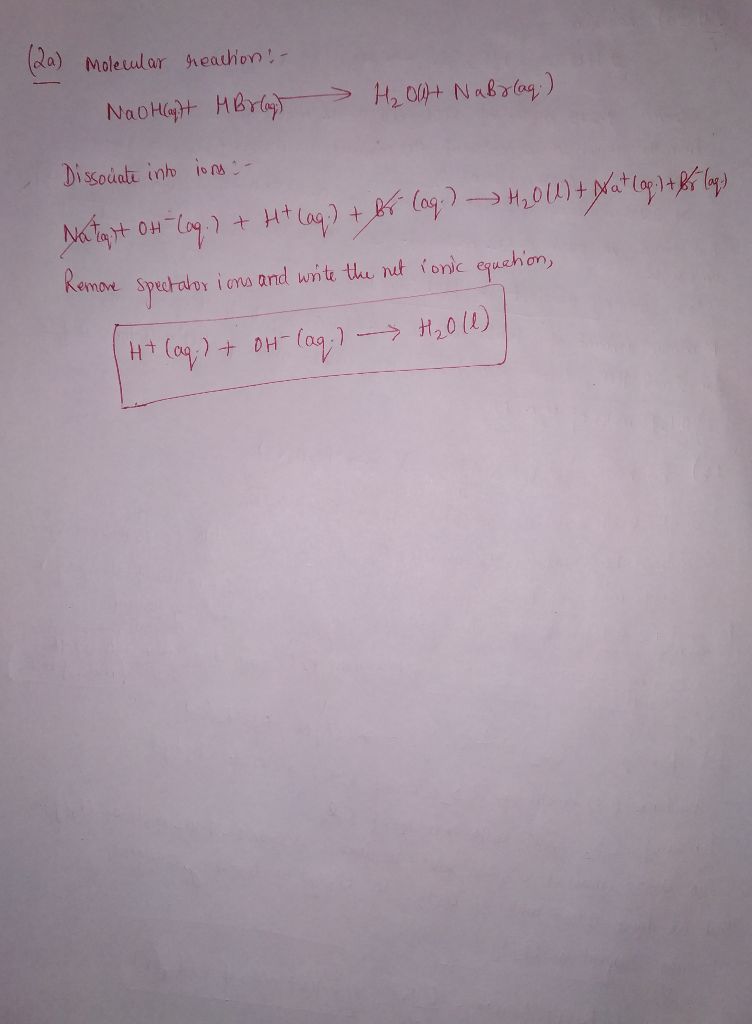##### Add Answer of: w4. 1a) For the following reaction, 0.275 moles of hydrochloric acid are mixed with 0.372 moles of oxygen gas. hydrochlo...
More Homework Help Questions Additional questions in this topic.